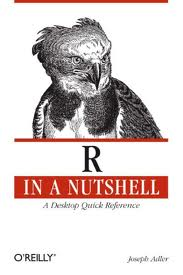Calculating characteristics such as median, mean,… of a subset of data is quite straightforward in R:

For a data set containing results from several “models”, a subset for the model “base” is created by

```base_only <- subset(all, all\$model == "base")
```

Then, the median of the variable “data” can be computed via

```median(base_only\$data)
```

## Fixed: Unable to plot a decent x-Axis in a time series plot using zoo

Here is the link to the original problem. Briefly, I was unable to plot a custom x-axis showing abbreviated months in a time series plot of a zoo object.

In the `plot.zoo()` function set

`xaxt = "n"`

to suppress plotting of the x-axis

Since I needed abbreviated months to be plotted as the x-axis, I loaded a csv file with the dates of the first of each month as follows and converted it to dates:

“month”
2004-12-01
2005-01-01
2005-02-01

(I included Dec 2004, because otherwise “Jan” would not be plotted)

`month < - read.csv("~/R/2005months.csv")`
`month\$month < - as.Date(month\$month, "%Y-%m-%d")`

After the `plot.zoo` command I added the following line –

`axis(1, month\$month, format(month\$month, "%b"))`

The solution was inspired by a post from Gabor Grothendieck on r-help. The original solution is still the only way to plot abbreviated months for time series plots of monthly averages.

## Boxplots without boxes

Let’s say you have several categories with multiple data points each that you would like to plot as individual points. Even if you have only a single point, the R graphics package will plot a line (without a box for lack of data). Overriding the default setting with e.g. pch = 1 does not help.

R’s `boxplot` function (or the `plot` function for that matter) – correctly – generates a boxplot for each category. If you would like to see individual points instead of boxes, the following code snippet could help by using the `points` function:

# Plot the boxplot as usual, but in white colour to make the boxes invisible, but keep the axes.
``` boxplot(m\$var ~ m\$cat, xlab = "Category", ylab = "Variable", border = "white")```

# Plot the data again into the same plot and customise the point shape, etc to your liking
``` points(m\$var ~ m\$cat, pch = 1) ```

Voila!

## R in a nutshellI got this book as a reference for my work with R and do like it. Just after browsing the chapters I already found some useful hints about loading and manipulating data, e.g., loading of fixed-width data files!

## More fun with boxplots

Here are a few more plotting options for boxplots:

Let’s start plotting the full set
`plot(b\$mod, b\$x)`

Plot labels for a subset in full set plot (label all points x < -1)
`text(subset(b\$mod, b\$x < -1), subset(b\$x, b\$x < -1), subset(b\$site, b\$x < -1), cex=0.6, pos=4, col="red")`

Plot subset with x > -1
`plot(subset(b\$mod, b\$x > -1), subset(b\$x, b\$x > -1))`

Plot horizontal gridlines
`grid(nx = NA, ny = NULL)`

## Converting vectors to numeric in mixed-type dataframe

Coercing variables of character and numeric type into a single dataframe yields all vectors to be defined as factors

`all <- data.frame(cbind(site, year, model, x, y, z))`

The following converts selected variables from “factor” back to “numeric”
```all\$x <- as.numeric(x) all\$y <- as.numeric(y) all\$z <- as.numeric(z)```

## Mapping points

Since I look at mercury concentrations at different measurement stations in North America, visualization using a map with values (of your favourite parameter) plotted as colour-coded circles is quite useful. After some trial & error, here is some very basic code to do this –

I have adapted a recipe from the Dept. of Geography, University of Oregon

```library(maps) library(maptools) library(RColorBrewer) library(classInt) library(gpclib) library(mapdata)```

# Define vector with the values that you would like to see plotted at desired lat/long. Your csv input file loaded as dataframe (Var) must feature the following columns (Site is optional, but useful for labeling)
Site,Para,Lat,Long
`plotvar <- Var\$Para`

# Define number of colours to be used in plot
`nclr <- 7`

# Define colour palette to be used
`plotclr <- brewer.pal(nclr,"RdPu")`

# Define colour intervals and colour code variable for plotting
```class <- classIntervals(plotvar, nclr, style = "pretty") colcode <- findColours(class, plotclr)```

# Plot the map with desired lat/long coordinates and data points with colour coding and legend
```map("worldHires", xlim = c(-125, -55), ylim = c(30, 83)) points(Var\$Long, Var\$Lat, pch = 16, col= colcode, cex = 2) legend("bottomright", legend = names(attr(colcode, "table")), fill = attr(colcode, "palette"), cex = 0.7, bty = "n")```

And here is the result:## Unable to plot a decent x-Axis in a time series plot using zoo

I use the R package zoo to plot a yearly time series of weekly averaged data. The problem is that my date variable (m.all\$date) contains week numbers and these are plotted as x-Axis. What I would rather like to to is plot abbreviated months.

I can suppress the x-Axis in the plot using `xaxt = "n"` in the `plot.zoo` command, but cannot define a suitable x-Axis that plot abbreviated months instead.

I tried several variations of the `axis.Date()` commands without luck!
``` # Create zoo object for time series plot z < - zoo(cbind(m.all\$obsTPM, m.all\$modTPM, m.all\$refTPM), m.all\$date) names(z) <- c("Observation","Model estimate", "Model reference") # Plot plot.zoo(z[, 1], type = "l", lwd = 1, col = "black", screens = c(1), xlab = "Date (2005)", ylim=c(m.all\$obsTPM,m.all\$modTPM, m.all\$refTPM), ylab = "Concentration (pg m-3)", main = "Alert TPM: Observations vs. Model Estimates, Weekly Means") lines(z[, 2], lty = 5, lwd = 1, col = "blue") lines(z[, 3], lty = 3, lwd = 1, col = "red") legend("topleft", lty = c(1,5,3), legend = colnames(z), bty = "n", col = c("black","blue","red"), lwd = 1) ```

## I am a useR

With the new project that I have taken on comes the requirement to deal with fairly large datasets. I do a comparison between mercury transport model estimations and observations and to handle the data I started using R – an open source language and environment for statistical computing and graphics!